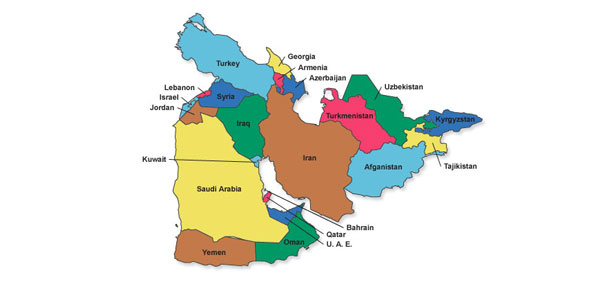# Fill In The Blank Questions For Middle East Capitals

25 Questions | Total Attempts: 86Settings• 1.
What is the capital of Kuwait?
• 2.
What is the capital of Tajakistan?
• 3.
What is the capital of Oman?
• 4.
What is the capital of Turkey?
• 5.
What is the capital of Cyprus?
• 6.
What is the capital of Pakistan?
• 7.
What is the capital of Saudi Arabia?
• 8.
What is the capital of Georgia?
• 9.
What is the capital of Syria?
• 10.
What is the capital of Uzbekistan?
• 11.
What is the capital of Israel?
• 12.
What is the capital of Iran?
• 13.
What is the capital of Jordan?
• 14.
What is the capital of Kyrgyztan?
• 15.
What is the capital of Yemen?
• 16.
What is the capital of Qatar?
• 17.
What is the capital of Turkmenistan?
• 18.
What is the capital of Iraq?
• 19.
What is the capital of Kazakhstan?
• 20.
What is the capital of U.A.E?
• 21.
What is the capital of Armenia?
• 22.
What is the capital of Lebanon?
• 23.
What is the capital of Afghanistan?
• 24.
What is the capital of Bahrain?
• 25.
What is the capital of Azerbaijan?
Related TopicsBack to top